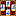###Scoring variations

#### Payments to winner – Korean scheme

This payoff scheme is used in Korean Style rule preset. If the winning tile is self-drawn, each loser pays according to the winner's final score. If the winning tile is a discard, only the discarder pays the winner's final score (other losers pay nothing).

Note that this scheme only simulates the Korean payoff scheme, which actually uses a system where a certain amount of points (faans) are added to the winner's final score only at the time of payments, depending on the way of going out. E.g., in a so called 1-5-10 scheme the payments are determined as follows (here it is assumed that the winner's final score is 3 points in all cases):

• If the winner goes out on a concealed self-drawn hand, 10 points are added to his final score. In Four Winds this is accomplished by giving 5 points (instead of 0 as in the original rules) for the winning and doubling it if the hand is fully concealed. So, in the original rules the winner gets 3 + 10 points = 13 points, whereas in Four Winds the bonus of 10 points is added already in the final score. This is paid by each loser so the winner's total payment would be 39.
• If the winner goes out self-drawn on a non-concealed hand, 5 points are added to this final score. In Four Winds this is accomplished by always giving 5 points (instead of 0) for winning. So, in the original rules the winner gets 3 (his winning score) + 5 points (for going out on a non-concealed self-drawn hand) = 8 points, which is paid by all losers, total payment being 24 points. In Four Winds the bonus of 5 points is added already in the final score, which is paid each loser.
• If the winner goes out on a discard (whether with already partially melded hand or with a concealed hand), 5 points are added to his final score. In Four Winds this is accomplished by always giving 5 points (instead of 0) for winning. So, in the original rules the winner gets 3 (his winning score) + 5 points (for going out on a discard) = 8 faans, which the discarder pays alone and doubled once, winner's total payment being 16 points. In Four Winds the bonus of 5 points is added already in the final score, and the discarder pays this sum doubled.
• Note that in Four Winds the bonus for Winning (5 points, doubled if a fully concealed hand) is ignored, when determining the minimum points of the hand.